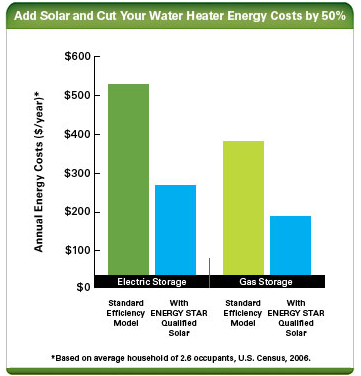# Cost and Energy Efficiency of a SWH

Estimating the Cost and Energy Efficiency of a Solar Water HeaterSolar water heaters are more efficient the gas or electric heaters.

Solar water heating systems usually cost more to purchase and install than conventional water heating systems. However, a solar water heater can usually save you money in the long run.

How much money you save depends on the following:
• The amount of hot water you use
• Your geographic location and solar resource
• Available financing and incentives
• The cost of conventional fuels (natural gas, oil, and electricity)
• The cost of the fuel you use for your backup water heating system, if you have one.

On average, if you install a solar water heater, your water heating bills should drop 50%–80%. Also, because the sun is free, you're protected from future fuel shortages and price hikes.

If you're building a new home or refinancing, the economics are even more attractive. Including the price of a solar water heater in a new 30-year mortgage usually amounts to between \$13 and \$20 per month. The federal income tax deduction for mortgage interest attributable to the solar system reduces that by about \$3–\$5 per month. So if your fuel savings are more than \$15 per month, the solar investment is profitable immediately. On a monthly basis, you're saving more than you're paying.

Determining Energy Efficiency of a Solar Water Heater
Use the solar energy factor (SEF) and solar fraction (SF) to determine a solar water heater's energy efficiency.

The solar energy factor is defined as the energy delivered by the system divided by the electrical or gas energy put into the system. The higher the number, the more energy efficient. Solar energy factors range from 1.0 to 11. Systems with solar energy factors of 2 or 3 are the most common.

Another solar water heater performance metric is the solar fraction. The solar fraction is the portion of the total conventional hot water heating load (delivered energy and tank standby losses). The higher the solar fraction, the greater the solar contribution to water heating, which reduces the energy required by the backup water heater. The solar fraction varies from 0 to 1.0. Typical solar factors are 0.5–0.75.

Don't choose a solar water heating system based solely on its energy efficiency. When selecting a solar water heater, it's also important to consider size and overall cost.

Calculating Annual Operating Cost
Before purchasing a solar water heating system, estimate the annual operating costs and compare several systems. This will help you determine the energy savings and payback period of investing in a more energy-efficient system, which will probably have a higher purchase price.

Before you can choose and compare the costs of various systems, you need to know the system size required for your home.

To estimate the annual operating cost of a solar water heating system, you need the following:
• The system's solar energy factor (SEF)
• The auxiliary tank fuel type (gas or electric) and costs (your local utility can provide current rates).
Then, use the following calculations.

WITH A GAS AUXILIARY TANK SYSTEM:
You need to know the unit cost of fuel by Btu (British thermal unit) or therm. (1 therm = 100,000 Btu)
365 × 41,045 ÷ SEF × Fuel Cost (Btu) = estimated annual cost of operation
OR
365 × 0.4105 ÷ SEF × Fuel Cost (therm) = estimated annual operating cost
Example: Assuming the SEF is 1.1 and the gas costs \$1.10/therm
365 × 0.4105 ÷ 1.1 × \$1.10 = \$149.83
The energy usage per day in the above equations is based on the DOE test procedure for hot water heaters, which assumes an incoming water temperature of 58°F, hot water temperature of 135°F, and total hot water production of 64.3 gallons per day, which is the average usage for a household of three people.

WITH AN ELECTRIC AUXILIARY TANK SYSTEM:
You need to know or convert the unit cost of electricity by kilowatt-hour (kWh).
365 × 12.03 kWh/day ÷ SEF × Electricity Cost (kWh)= estimated annual operating cost
Example: Assuming the SEF is 2.0 and the electricity costs \$0.08/kWh
365 X 12.03 ÷ 2.0 X \$0.08 = \$175.64

Comparing Costs and Determining Payback
Once you know the purchase and annual operating costs of the solar water heating systems you want to compare, you can find the costs associated with conventional water heating systems and compare the two.
Use the table and calculations below to compare two solar water heating systems and determine the cost savings and payback of the more energy-efficient system model.
 System Models System Price SEF Estimated Annual Operating Cost System Model A System Model B (higher SEF) Additional cost of more efficient model (Model B) Price of System Model B  - Price of System Model A = \$Additional Cost of Model B Estimated annual operating cost savings (System Model B) System Model B Annual Operating Cost - System Model A Annual Operating Cost = \$Model B's Cost Savings Per Year Payback period for Model B \$Additional Cost of Model B/\$Model B's Cost Savings Per Year = Payback period/years
Example:
Comparison of two solar water heating system models with electric backup systems and electricity costs of \$0.08/kWh.
 System Models System Price SEF Estimated Annual Operating Cost System Model A \$1,060 2.0 \$176 System Model B \$1,145 2.9 \$121 Additional cost of more efficient model (Model B) \$1,145-\$1,060 = \$85 Estimated annual operating cost savings (Model B) \$176-\$120 = \$56 per year Payback period for Model B \$85/\$56 per year = 1.5 years
Other Costs
When comparing solar water heating systems, you should also consider installation and maintenance costs. Some systems might cost more to install and maintain.
Consult the manufacturer(s) and a qualified contractor to help estimate these costs. These costs will vary among system types and sometimes even from model to model.